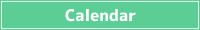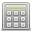<< < Dec. 2023 > >>
SunMonTueWedThuFriSat
26 27 28 29 30 1 2
3 4 5 6 7 8 9
10 11 12 13 14 15 16
17 18 19 20 21 22 23
24 25 26 27 28 29 30
31 1 2 3 4 5 6## What is total daily energy expenditure (TDEE)?

Total daily energy expenditure (TDEE) is the total amount of energy a person consumes in a day.

TDEE stands for "Total Daily Energy Expenditure".

This depends on your basal metabolic rate and how much exercise you do each day.

The basal metabolic rate is the amount of energy consumed even when a person is not doing anything, and is the minimum amount of energy required to live.

The basal metabolic rate changes with age, and is calculated here using the Harris-Benedict equation from gender, age, height, and weight.

Total daily energy expenditure (TDEE) is calculated by adding the energy consumed by daily physical activity to this basal metabolic rate.

Here, this basal metabolic rate is multiplied by a multiplier for each activity level.

## Formula for calculating basal metabolic rate (BMR)

The basal metabolic rate is calculated using the following formula.

Male: BMR = 88.362 + (13.397 x Body weight(kg)) + (4.799 x Height(cm)) - (5.677 x Age(years old))

Female: BMR = 447.593 + (9.247 x Body weight(kg)) + (3.098 x Height(cm)) - (4.330 x Age(years old))

Harris-Benedict equation (improved version)

## Total daily energy expenditure (TDEE) formula

Total daily energy expenditure (TDEE) is calculated by the following formula using the basal metabolic rate obtained from the basal metabolic rate (BMR) formula.

Rarely exercise: Basal metabolic rate x 1.2

light exercise: Basal metabolic rate x 1.375

Moderate exercise: Basal metabolic rate x 1.55

Strenuous exercise: Basal metabolic rate x 1.725

Very strenuous exercise: Basal metabolic rate x 1.9

###Simple Calculator

Save results in a temporary